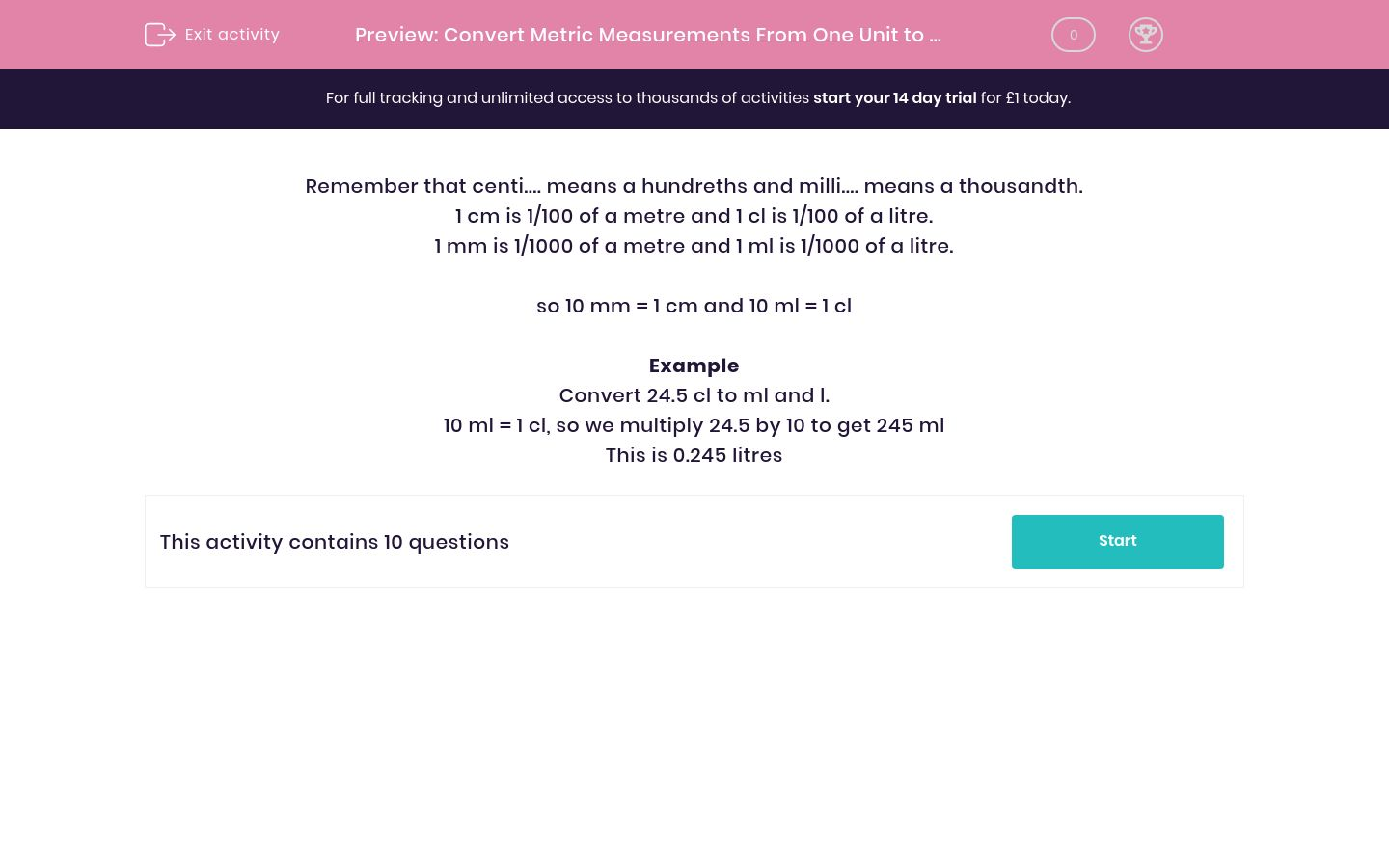# Convert Metric Measurements From One Unit to Another

In this worksheet, students convert metric measurements from one unit to another.Key stage:  KS 2

Curriculum topic:   Maths and Numerical Reasoning

Curriculum subtopic:   Metric & Imperial

Difficulty level:### QUESTION 1 of 10

Remember that centi.... means a hundreths and milli.... means a thousandth.

1 cm is 1/100 of a metre and 1 cl is 1/100 of a litre.

1 mm is 1/1000 of a metre and 1 ml is 1/1000 of a litre.

so 10 mm = 1 cm and 10 ml = 1 cl

Example

Convert 24.5 cl to ml and l.

10 ml = 1 cl, so we multiply 24.5 by 10 to get 245 ml

This is 0.245 litres

Convert the following measurement into the given unit.

8.3 g =  ______________ mg

Convert the following measurement into the given unit.

6.8 cl =  ______________ ml

Convert the following measurement into the given unit.

5.6 km =  ______________ m

Convert the following measurement into the given unit.

26.3 cl =  ______________ ml

Convert the following measurement into the given unit.

4.7 cl =  ______________ ml

Convert the following measurement into the given unit.

67242 cm =  ______________ km

Convert the following measurement into the given unit.

41358 cl =  ______________ litres

Convert the following measurement into the given unit.

8.4 g =  ______________ mg

Convert the following measurement into the given unit.

19173 cm =  ______________ m

Convert the following measurement into the given unit.

82028 cl =  ______________ litres

• Question 1

Convert the following measurement into the given unit.

8.3 g =  ______________ mg

8300
• Question 2

Convert the following measurement into the given unit.

6.8 cl =  ______________ ml

68
• Question 3

Convert the following measurement into the given unit.

5.6 km =  ______________ m

5600
• Question 4

Convert the following measurement into the given unit.

26.3 cl =  ______________ ml

263
• Question 5

Convert the following measurement into the given unit.

4.7 cl =  ______________ ml

47
• Question 6

Convert the following measurement into the given unit.

67242 cm =  ______________ km

0.67242
• Question 7

Convert the following measurement into the given unit.

41358 cl =  ______________ litres

413.58
• Question 8

Convert the following measurement into the given unit.

8.4 g =  ______________ mg

8400
• Question 9

Convert the following measurement into the given unit.

19173 cm =  ______________ m

191.73
• Question 10

Convert the following measurement into the given unit.

82028 cl =  ______________ litres

820.28
---- OR ----

Sign up for a £1 trial so you can track and measure your child's progress on this activity.

### What is EdPlace?

We're your National Curriculum aligned online education content provider helping each child succeed in English, maths and science from year 1 to GCSE. With an EdPlace account you’ll be able to track and measure progress, helping each child achieve their best. We build confidence and attainment by personalising each child’s learning at a level that suits them.

Get started